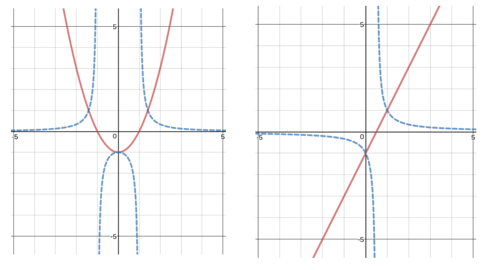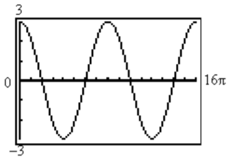Match Fishtank is now Fishtank Learning!

# Unit Circle and Trigonometric Functions

## Objective

Identify equations and graphs of all six trigonometric functions.

## Common Core Standards

### Core Standards

?

• F.BF.B.3 — Identify the effect on the graph of replacing f(x) by f(x) + k, k f(x), f(kx), and f(x + k) for specific values of k (both positive and negative); find the value of k given the graphs. Experiment with cases and illustrate an explanation of the effects on the graph using technology. Include recognizing even and odd functions from their graphs and algebraic expressions for them.

• F.TF.A.3 — Use special triangles to determine geometrically the values of sine, cosine, tangent for π/3, π/4 and π/6, and use the unit circle to express the values of sine, cosine, and tangent for π-x, π+x, and 2π-x in terms of their values for x, where x is any real number.

• F.TF.B.5 — Choose trigonometric functions to model periodic phenomena with specified amplitude, frequency, and midline. Modeling is best interpreted not as a collection of isolated topics but in relation to other standards. Making mathematical models is a Standard for Mathematical Practice, and specific modeling standards appear throughout the high school standards indicated by a star symbol (★). The star symbol sometimes appears on the heading for a group of standards; in that case, it should be understood to apply to all standards in that group.

## Criteria for Success

?

1. Use features of trigonometric functions to identify corresponding graphs and descriptions.
2. Establish reasoning for why an equation matches with one graph but not another.
3. Recognize secant, cosecant, and cotangent graphs as reciprocals of the other trig functions.
4. Critique reasoning of others and describe flawed reasoning when matching features, functions, and graphs.

## Anchor Problems

?

### Problem 1

Show students the word doc linked in the blog post Sinusoidal Sort by Chris Hunter.

Ask, "Which functions, descriptions, and graphs match together?"

#### References

Reflections in the Why Sinusoidal Sort

Sinusoidal Sort by Chris Hunter made available on Reflection in the Why under a CC BY-NC 4.0 license. Accessed Feb. 26, 2018, 4:10 p.m..

### Problem 2

In each graph below, a function is graphed as a solid line and its reciprocal is graphed as a dotted line. What features of each function can you connect to its reciprocal?Based on what you notice, sketch graphs of ${y=\mathrm{sec}x}$ and ${y=\mathrm{csc}x}$.

## Problem Set

?

The following resources include problems and activities aligned to the objective of the lesson that can be used to create your own problem set.

• Include problems recognizing secant and cosecant graphs. Students don’t need to sketch graphs themselves, but they should recognize them, connect them with sine and cosine graphs, and understand the reciprocal relationship.
• Include problems connecting transformations of cosecant and secant functions to transformations of sine and cosine functions.
• Includes review of graphing all trigonometric functions.
• Include problems writing equations and sketching graphs from a list of characteristics.

?

Which one of the equations below matches the graph?A)    ${y=-3\mathrm{sin}(4x)}$

B)    ${y=3\mathrm{cos}(4x)}$

C)    ${y=3\mathrm{sin}\left(\frac{1}{4}x\right)}$

D)    ${y=3\mathrm{cos}\left(\frac{1}{4}x\right)}$